# mne.time_frequency.AverageTFR¶

class mne.time_frequency.AverageTFR(info, data, times, freqs, nave, comment=None, method=None, verbose=None)[source]

Container for Time-Frequency data.

Can for example store induced power at sensor level or inter-trial coherence.

Parameters
infoInfo

The measurement info.

datandarray, shape (n_channels, n_freqs, n_times)

The data.

timesndarray, shape (n_times,)

The time values in seconds.

freqsndarray, shape (n_freqs,)

The frequencies in Hz.

naveint

The number of averaged TFRs.

comment

Comment on the data, e.g., the experimental condition.

method

Comment on the method used to compute the data, e.g., morlet wavelet.

verbose

If not None, override default verbose level (see mne.verbose() and Logging documentation for more). If used, it should be passed as a keyword-argument only.

Attributes
infoinstance of Info

Measurement info.

ch_nameslist

Channel names.

naveint

Number of averaged epochs.

datandarray, shape (n_channels, n_freqs, n_times)

The data array.

timesndarray, shape (n_times,)

The time values in seconds.

freqsndarray, shape (n_freqs,)

The frequencies in Hz.

commentstr

Comment on dataset. Can be the condition.

method

Comment on the method used to compute the data, e.g., morlet wavelet.

Methods

 __add__(tfr) Add instances. __contains__(ch_type) Check channel type membership. Hash the object. Multiply source instances. __sub__(tfr) Subtract instances. add_channels(add_list[, force_update_info]) Append new channels to the instance. apply_baseline(baseline[, mode, verbose]) Baseline correct the data. Return a copy of the instance. crop([tmin, tmax, fmin, fmax, include_tmax]) Crop data to a given time interval in place. drop_channels(ch_names) Drop channel(s). get_channel_types([picks, unique, only_data_chs]) Get a list of channel type for each channel. Get a DigMontage from instance. pick(picks[, exclude]) Pick a subset of channels. pick_channels(ch_names[, ordered]) Pick some channels. pick_types([meg, eeg, stim, eog, ecg, emg, …]) Pick some channels by type and names. plot([picks, baseline, mode, tmin, tmax, …]) Plot TFRs as a two-dimensional image(s). plot_joint([timefreqs, picks, baseline, …]) Plot TFRs as a two-dimensional image with topomaps. plot_topo([picks, baseline, mode, tmin, …]) Plot TFRs in a topography with images. plot_topomap([tmin, tmax, fmin, fmax, …]) Plot topographic maps of time-frequency intervals of TFR data. reorder_channels(ch_names) Reorder channels. save(fname[, overwrite]) Save TFR object to hdf5 file.
__add__(tfr)[source]

__contains__(ch_type)[source]

Check channel type membership.

Parameters
ch_typestr

Channel type to check for. Can be e.g. ‘meg’, ‘eeg’, ‘stim’, etc.

Returns
inbool

Whether or not the instance contains the given channel type.

Examples

Channel type membership can be tested as:

>>> 'meg' in inst
True
>>> 'seeg' in inst
False

__hash__()[source]

Hash the object.

Returns
hashint

The hash

__mul__(a)[source]

Multiply source instances.

__sub__(tfr)[source]

Subtract instances.

add_channels(add_list, force_update_info=False)[source]

Append new channels to the instance.

Parameters
add_listlist

A list of objects to append to self. Must contain all the same type as the current object.

force_update_infobool

If True, force the info for objects to be appended to match the values in self. This should generally only be used when adding stim channels for which important metadata won’t be overwritten.

New in version 0.12.

Returns
instinstance of Raw, Epochs, or Evoked

The modified instance.

Notes

If self is a Raw instance that has been preloaded into a numpy.memmap instance, the memmap will be resized.

apply_baseline(baseline, mode='mean', verbose=None)[source]

Baseline correct the data.

Parameters
baselinearray_like, shape (2,)

The time interval to apply rescaling / baseline correction. If None do not apply it. If baseline is (a, b) the interval is between “a (s)” and “b (s)”. If a is None the beginning of the data is used and if b is None then b is set to the end of the interval. If baseline is equal to (None, None) all the time interval is used.

mode‘mean’ | ‘ratio’ | ‘logratio’ | ‘percent’ | ‘zscore’ | ‘zlogratio’

Perform baseline correction by

• subtracting the mean of baseline values (‘mean’)

• dividing by the mean of baseline values (‘ratio’)

• dividing by the mean of baseline values and taking the log (‘logratio’)

• subtracting the mean of baseline values followed by dividing by the mean of baseline values (‘percent’)

• subtracting the mean of baseline values and dividing by the standard deviation of baseline values (‘zscore’)

• dividing by the mean of baseline values, taking the log, and dividing by the standard deviation of log baseline values (‘zlogratio’)

verbose

If not None, override default verbose level (see mne.verbose() and Logging documentation for more). If used, it should be passed as a keyword-argument only. Defaults to self.verbose.

Returns
instinstance of AverageTFR

The modified instance.

property ch_names

Channel names.

property compensation_grade

copy()[source]

Return a copy of the instance.

Returns
copyinstance of EpochsTFR | instance of AverageTFR

A copy of the instance.

crop(tmin=None, tmax=None, fmin=None, fmax=None, include_tmax=True)[source]

Crop data to a given time interval in place.

Parameters
tmin

Start time of selection in seconds.

tmax

End time of selection in seconds.

fmin

Lowest frequency of selection in Hz.

New in version 0.18.0.

fmax

Highest frequency of selection in Hz.

New in version 0.18.0.

include_tmaxbool

If True (default), include tmax. If False, exclude tmax (similar to how Python indexing typically works).

New in version 0.19.

Returns
instinstance of AverageTFR

The modified instance.

drop_channels(ch_names)[source]

Drop channel(s).

Parameters
ch_names

Iterable (e.g. list) of channel name(s) or channel name to remove.

Returns
instinstance of Raw, Epochs, or Evoked

The modified instance.

Notes

New in version 0.9.0.

get_channel_types(picks=None, unique=False, only_data_chs=False)[source]

Get a list of channel type for each channel.

Parameters
picks

Channels to include. Slices and lists of integers will be interpreted as channel indices. In lists, channel type strings (e.g., ['meg', 'eeg']) will pick channels of those types, channel name strings (e.g., ['MEG0111', 'MEG2623'] will pick the given channels. Can also be the string values “all” to pick all channels, or “data” to pick data channels. None (default) will pick all channels.

uniquebool

Whether to return only unique channel types. Default is False.

only_data_chsbool

Whether to ignore non-data channels. Default is False.

Returns
channel_typeslist

The channel types.

get_montage()[source]

Get a DigMontage from instance.

Returns
montage

A montage containing channel positions. If str or DigMontage is specified, the channel info will be updated with the channel positions. Default is None. See also the documentation of mne.channels.DigMontage for more information.

pick(picks, exclude=())[source]

Pick a subset of channels.

Parameters
picks

Channels to include. Slices and lists of integers will be interpreted as channel indices. In lists, channel type strings (e.g., ['meg', 'eeg']) will pick channels of those types, channel name strings (e.g., ['MEG0111', 'MEG2623'] will pick the given channels. Can also be the string values “all” to pick all channels, or “data” to pick data channels. None (default) will pick all channels.

exclude

Set of channels to exclude, only used when picking based on types (e.g., exclude=”bads” when picks=”meg”).

Returns
instinstance of Raw, Epochs, or Evoked

The modified instance.

pick_channels(ch_names, ordered=False)[source]

Pick some channels.

Parameters
ch_nameslist

The list of channels to select.

orderedbool

If True (default False), ensure that the order of the channels in the modified instance matches the order of ch_names.

New in version 0.20.0.

Returns
instinstance of Raw, Epochs, or Evoked

The modified instance.

Notes

The channel names given are assumed to be a set, i.e. the order does not matter. The original order of the channels is preserved. You can use reorder_channels to set channel order if necessary.

New in version 0.9.0.

pick_types(meg=None, eeg=False, stim=False, eog=False, ecg=False, emg=False, ref_meg='auto', misc=False, resp=False, chpi=False, exci=False, ias=False, syst=False, seeg=False, dipole=False, gof=False, bio=False, ecog=False, fnirs=False, csd=False, include=(), exclude='bads', selection=None, verbose=None)[source]

Pick some channels by type and names.

Parameters
meg

If True include MEG channels. If string it can be ‘mag’, ‘grad’, ‘planar1’ or ‘planar2’ to select only magnetometers, all gradiometers, or a specific type of gradiometer.

eegbool

If True include EEG channels.

stimbool

If True include stimulus channels.

eogbool

If True include EOG channels.

ecgbool

If True include ECG channels.

emgbool

If True include EMG channels.

ref_meg

If True include CTF / 4D reference channels. If ‘auto’, reference channels are included if compensations are present and meg is not False. Can also be the string options for the meg parameter.

miscbool

If True include miscellaneous analog channels.

respbool

If True include response-trigger channel. For some MEG systems this is separate from the stim channel.

chpibool

If True include continuous HPI coil channels.

excibool

Flux excitation channel used to be a stimulus channel.

iasbool

Internal Active Shielding data (maybe on Triux only).

systbool

System status channel information (on Triux systems only).

seegbool

Stereotactic EEG channels.

dipolebool

Dipole time course channels.

gofbool

Dipole goodness of fit channels.

biobool

Bio channels.

ecogbool

Electrocorticography channels.

fnirs

Functional near-infrared spectroscopy channels. If True include all fNIRS channels. If False (default) include none. If string it can be ‘hbo’ (to include channels measuring oxyhemoglobin) or ‘hbr’ (to include channels measuring deoxyhemoglobin).

csdbool

EEG-CSD channels.

include

List of additional channels to include. If empty do not include any.

exclude

List of channels to exclude. If ‘bads’ (default), exclude channels in info['bads'].

selection

Restrict sensor channels (MEG, EEG) to this list of channel names.

verbose

If not None, override default verbose level (see mne.verbose() and Logging documentation for more). If used, it should be passed as a keyword-argument only. Defaults to self.verbose.

Returns
instinstance of Raw, Epochs, or Evoked

The modified instance.

Notes

New in version 0.9.0.

plot(picks=None, baseline=None, mode='mean', tmin=None, tmax=None, fmin=None, fmax=None, vmin=None, vmax=None, cmap='RdBu_r', dB=False, colorbar=True, show=True, title=None, axes=None, layout=None, yscale='auto', mask=None, mask_style=None, mask_cmap='Greys', mask_alpha=0.1, combine=None, exclude=[], verbose=None)[source]

Plot TFRs as a two-dimensional image(s).

Parameters
picks

Channels to include. Slices and lists of integers will be interpreted as channel indices. In lists, channel type strings (e.g., ['meg', 'eeg']) will pick channels of those types, channel name strings (e.g., ['MEG0111', 'MEG2623'] will pick the given channels. Can also be the string values “all” to pick all channels, or “data” to pick data channels. None (default) will pick good data channels.

baselineNone (default) or tuple, shape (2,)

The time interval to apply baseline correction. If None do not apply it. If baseline is (a, b) the interval is between “a (s)” and “b (s)”. If a is None the beginning of the data is used and if b is None then b is set to the end of the interval. If baseline is equal to (None, None) all the time interval is used.

mode‘mean’ | ‘ratio’ | ‘logratio’ | ‘percent’ | ‘zscore’ | ‘zlogratio’

Perform baseline correction by

• subtracting the mean of baseline values (‘mean’)

• dividing by the mean of baseline values (‘ratio’)

• dividing by the mean of baseline values and taking the log (‘logratio’)

• subtracting the mean of baseline values followed by dividing by the mean of baseline values (‘percent’)

• subtracting the mean of baseline values and dividing by the standard deviation of baseline values (‘zscore’)

• dividing by the mean of baseline values, taking the log, and dividing by the standard deviation of log baseline values (‘zlogratio’)

tmin

The first time instant to display. If None the first time point available is used.

tmax

The last time instant to display. If None the last time point available is used.

fmin

The first frequency to display. If None the first frequency available is used.

fmax

The last frequency to display. If None the last frequency available is used.

vmin

The minimum value an the color scale. If vmin is None, the data minimum value is used.

vmax

The maximum value an the color scale. If vmax is None, the data maximum value is used.

cmapmatplotlib colormap | ‘interactive’ | (colormap, bool)

The colormap to use. If tuple, the first value indicates the colormap to use and the second value is a boolean defining interactivity. In interactive mode the colors are adjustable by clicking and dragging the colorbar with left and right mouse button. Left mouse button moves the scale up and down and right mouse button adjusts the range. Hitting space bar resets the range. Up and down arrows can be used to change the colormap. If ‘interactive’, translates to (‘RdBu_r’, True). Defaults to ‘RdBu_r’.

Warning

Interactive mode works smoothly only for a small amount of images.

dBbool

If True, 10*log10 is applied to the data to get dB.

colorbarbool

If true, colorbar will be added to the plot. For user defined axes, the colorbar cannot be drawn. Defaults to True.

showbool

Call pyplot.show() at the end.

titlestr | ‘auto’ | None

String for title. Defaults to None (blank/no title). If ‘auto’, automatically create a title that lists up to 6 of the channels used in the figure.

axesinstance of Axes | list | None

The axes to plot to. If list, the list must be a list of Axes of the same length as the number of channels. If instance of Axes, there must be only one channel plotted.

layout

Layout instance specifying sensor positions. Used for interactive plotting of topographies on rectangle selection. If possible, the correct layout is inferred from the data.

yscale‘auto’ (default) | ‘linear’ | ‘log’

The scale of y (frequency) axis. ‘linear’ gives linear y axis, ‘log’ leads to log-spaced y axis and ‘auto’ detects if frequencies are log-spaced and only then sets the y axis to ‘log’.

New in version 0.14.0.

An array of booleans of the same shape as the data. Entries of the data that correspond to False in the mask are plotted transparently. Useful for, e.g., masking for statistical significance.

New in version 0.16.0.

mask_styleNone | ‘both’ | ‘contour’ | ‘mask’

If mask is not None: if 'contour', a contour line is drawn around the masked areas (True in mask). If 'mask', entries not True in mask are shown transparently. If 'both', both a contour and transparency are used. If None, defaults to 'both' if mask is not None, and is ignored otherwise.

New in version 0.17.

mask_cmapmatplotlib colormap | (colormap, bool) | ‘interactive’

The colormap chosen for masked parts of the image (see below), if mask is not None. If None, cmap is reused. Defaults to 'Greys'. Not interactive. Otherwise, as cmap.

New in version 0.17.

mask_alphafloat

A float between 0 and 1. If mask is not None, this sets the alpha level (degree of transparency) for the masked-out segments. I.e., if 0, masked-out segments are not visible at all. Defaults to 0.1.

New in version 0.16.0.

combine‘mean’ | ‘rms’ | None

Type of aggregation to perform across selected channels. If None, plot one figure per selected channel.

excludelist of str | ‘bads’

Channels names to exclude from being shown. If ‘bads’, the bad channels are excluded. Defaults to an empty list.

verbose

If not None, override default verbose level (see mne.verbose() and Logging documentation for more). If used, it should be passed as a keyword-argument only. Defaults to self.verbose.

Returns
figmatplotlib.figure.Figure

The figure containing the topography.

Examples using plot: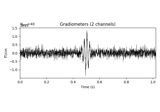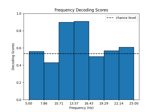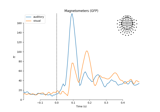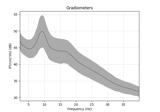plot_joint(timefreqs=None, picks=None, baseline=None, mode='mean', tmin=None, tmax=None, fmin=None, fmax=None, vmin=None, vmax=None, cmap='RdBu_r', dB=False, colorbar=True, show=True, title=None, yscale='auto', combine='mean', exclude=[], topomap_args=None, image_args=None, verbose=None)[source]

Plot TFRs as a two-dimensional image with topomaps.

Parameters
timefreqs

The time-frequency point(s) for which topomaps will be plotted. See Notes.

picks

Channels to include. Slices and lists of integers will be interpreted as channel indices. In lists, channel type strings (e.g., ['meg', 'eeg']) will pick channels of those types, channel name strings (e.g., ['MEG0111', 'MEG2623'] will pick the given channels. Can also be the string values “all” to pick all channels, or “data” to pick data channels. None (default) will pick good data channels.

baselineNone (default) or tuple of length 2

The time interval to apply baseline correction. If None do not apply it. If baseline is (a, b) the interval is between “a (s)” and “b (s)”. If a is None, the beginning of the data is used. If b is None, then b is set to the end of the interval. If baseline is equal to (None, None), the entire time interval is used.

mode

If str, must be one of ‘ratio’, ‘zscore’, ‘mean’, ‘percent’, ‘logratio’ and ‘zlogratio’. Do baseline correction with ratio (power is divided by mean power during baseline) or zscore (power is divided by standard deviation of power during baseline after subtracting the mean, power = [power - mean(power_baseline)] / std(power_baseline)), mean simply subtracts the mean power, percent is the same as applying ratio then mean, logratio is the same as mean but then rendered in log-scale, zlogratio is the same as zscore but data is rendered in log-scale first. If None no baseline correction is applied.

tmin

The first time instant to display. If None the first time point available is used.

tmax

The last time instant to display. If None the last time point available is used.

fmin

The first frequency to display. If None the first frequency available is used.

fmax

The last frequency to display. If None the last frequency available is used.

vmin

The minimum value of the color scale for the image (for topomaps, see topomap_args). If vmin is None, the data absolute minimum value is used.

vmax

The maximum value of the color scale for the image (for topomaps, see topomap_args). If vmax is None, the data absolute maximum value is used.

cmapmatplotlib colormap

The colormap to use.

dBbool

If True, 10*log10 is applied to the data to get dB.

colorbarbool

If true, colorbar will be added to the plot (relating to the topomaps). For user defined axes, the colorbar cannot be drawn. Defaults to True.

showbool

Call pyplot.show() at the end.

title

String for title. Defaults to None (blank/no title).

yscale‘auto’ (default) | ‘linear’ | ‘log’

The scale of y (frequency) axis. ‘linear’ gives linear y axis, ‘log’ leads to log-spaced y axis and ‘auto’ detects if frequencies are log-spaced and only then sets the y axis to ‘log’.

combine‘mean’ | ‘rms’

Type of aggregation to perform across selected channels.

excludelist of str | ‘bads’

Channels names to exclude from being shown. If ‘bads’, the bad channels are excluded. Defaults to an empty list, i.e., [].

topomap_args

A dict of kwargs that are forwarded to mne.viz.plot_topomap() to style the topomaps. axes and show are ignored. If times is not in this dict, automatic peak detection is used. Beyond that, if None, no customizable arguments will be passed. Defaults to None.

image_args

A dict of kwargs that are forwarded to AverageTFR.plot() to style the image. axes and show are ignored. Beyond that, if None, no customizable arguments will be passed. Defaults to None.

verbose

If not None, override default verbose level (see mne.verbose() and Logging documentation for more). If used, it should be passed as a keyword-argument only. Defaults to self.verbose.

Returns
figmatplotlib.figure.Figure

The figure containing the topography.

Notes

timefreqs has three different modes: tuples, dicts, and auto. For (list of) tuple(s) mode, each tuple defines a pair (time, frequency) in s and Hz on the TFR plot. For example, to look at 10 Hz activity 1 second into the epoch and 3 Hz activity 300 msec into the epoch,

timefreqs=((1, 10), (.3, 3))


If provided as a dictionary, (time, frequency) tuples are keys and (time_window, frequency_window) tuples are the values - indicating the width of the windows (centered on the time and frequency indicated by the key) to be averaged over. For example,

timefreqs={(1, 10): (0.1, 2)}


would translate into a window that spans 0.95 to 1.05 seconds, as well as 9 to 11 Hz. If None, a single topomap will be plotted at the absolute peak across the time-frequency representation.

New in version 0.16.0.

Examples using plot_joint:plot_topo(picks=None, baseline=None, mode='mean', tmin=None, tmax=None, fmin=None, fmax=None, vmin=None, vmax=None, layout=None, cmap='RdBu_r', title=None, dB=False, colorbar=True, layout_scale=0.945, show=True, border='none', fig_facecolor='k', fig_background=None, font_color='w', yscale='auto', verbose=None)[source]

Plot TFRs in a topography with images.

Parameters
picks

Channels to include. Slices and lists of integers will be interpreted as channel indices. In lists, channel type strings (e.g., ['meg', 'eeg']) will pick channels of those types, channel name strings (e.g., ['MEG0111', 'MEG2623'] will pick the given channels. Can also be the string values “all” to pick all channels, or “data” to pick data channels. None (default) will pick good data channels.

baselineNone (default) or tuple of length 2

The time interval to apply baseline correction. If None do not apply it. If baseline is (a, b) the interval is between “a (s)” and “b (s)”. If a is None the beginning of the data is used and if b is None then b is set to the end of the interval. If baseline is equal to (None, None) all the time interval is used.

mode‘mean’ | ‘ratio’ | ‘logratio’ | ‘percent’ | ‘zscore’ | ‘zlogratio’

Perform baseline correction by

• subtracting the mean of baseline values (‘mean’)

• dividing by the mean of baseline values (‘ratio’)

• dividing by the mean of baseline values and taking the log (‘logratio’)

• subtracting the mean of baseline values followed by dividing by the mean of baseline values (‘percent’)

• subtracting the mean of baseline values and dividing by the standard deviation of baseline values (‘zscore’)

• dividing by the mean of baseline values, taking the log, and dividing by the standard deviation of log baseline values (‘zlogratio’)

tmin

The first time instant to display. If None the first time point available is used.

tmax

The last time instant to display. If None the last time point available is used.

fmin

The first frequency to display. If None the first frequency available is used.

fmax

The last frequency to display. If None the last frequency available is used.

vmin

The minimum value of the color scale. If vmin is None, the data minimum value is used.

vmax

The maximum value of the color scale. If vmax is None, the data maximum value is used.

layout

Layout instance specifying sensor positions. If possible, the correct layout is inferred from the data.

cmapmatplotlib colormap | str

The colormap to use. Defaults to ‘RdBu_r’.

titlestr

Title of the figure.

dBbool

If True, 10*log10 is applied to the data to get dB.

colorbarbool

If true, colorbar will be added to the plot.

layout_scalefloat

Scaling factor for adjusting the relative size of the layout on the canvas.

showbool

Call pyplot.show() at the end.

borderstr

Matplotlib borders style to be used for each sensor plot.

fig_facecolorcolor

The figure face color. Defaults to black.

fig_background

A background image for the figure. This must be a valid input to matplotlib.pyplot.imshow. Defaults to None.

font_colorcolor

The color of tick labels in the colorbar. Defaults to white.

yscale‘auto’ (default) | ‘linear’ | ‘log’

The scale of y (frequency) axis. ‘linear’ gives linear y axis, ‘log’ leads to log-spaced y axis and ‘auto’ detects if frequencies are log-spaced and only then sets the y axis to ‘log’.

verbose

If not None, override default verbose level (see mne.verbose() and Logging documentation for more). If used, it should be passed as a keyword-argument only.

Returns
figmatplotlib.figure.Figure

The figure containing the topography.

Examples using plot_topo: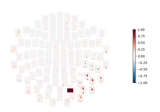plot_topomap(tmin=None, tmax=None, fmin=None, fmax=None, ch_type=None, baseline=None, mode='mean', vmin=None, vmax=None, cmap=None, sensors=True, colorbar=True, unit=None, res=64, size=2, cbar_fmt='%1.1e', show_names=False, title=None, axes=None, show=True, outlines='head', contours=6, sphere=None)[source]

Plot topographic maps of time-frequency intervals of TFR data.

Parameters
tmin

The first time instant to display. If None the first time point available is used.

tmax

The last time instant to display. If None the last time point available is used.

fmin

The first frequency to display. If None the first frequency available is used.

fmax

The last frequency to display. If None the last frequency available is used.

ch_type‘mag’ | ‘grad’ | ‘planar1’ | ‘planar2’ | ‘eeg’ | None

The channel type to plot. For ‘grad’, the gradiometers are collected in pairs and the RMS for each pair is plotted. If None, then first available channel type from order given above is used. Defaults to None.

baselinetuple or list of length 2

The time interval to apply rescaling / baseline correction. If None do not apply it. If baseline is (a, b) the interval is between “a (s)” and “b (s)”. If a is None the beginning of the data is used and if b is None then b is set to the end of the interval. If baseline is equal to (None, None) all the time interval is used.

mode‘mean’ | ‘ratio’ | ‘logratio’ | ‘percent’ | ‘zscore’ | ‘zlogratio’

Perform baseline correction by

• subtracting the mean of baseline values (‘mean’)

• dividing by the mean of baseline values (‘ratio’)

• dividing by the mean of baseline values and taking the log (‘logratio’)

• subtracting the mean of baseline values followed by dividing by the mean of baseline values (‘percent’)

• subtracting the mean of baseline values and dividing by the standard deviation of baseline values (‘zscore’)

• dividing by the mean of baseline values, taking the log, and dividing by the standard deviation of log baseline values (‘zlogratio’)

vmin

The value specifying the lower bound of the color range. If None, and vmax is None, -vmax is used. Else np.min(data) or in case data contains only positive values 0. If callable, the output equals vmin(data). Defaults to None.

vmax

The value specifying the upper bound of the color range. If None, the maximum value is used. If callable, the output equals vmax(data). Defaults to None.

cmapmatplotlib colormap | (colormap, bool) | ‘interactive’ | None

Colormap to use. If tuple, the first value indicates the colormap to use and the second value is a boolean defining interactivity. In interactive mode the colors are adjustable by clicking and dragging the colorbar with left and right mouse button. Left mouse button moves the scale up and down and right mouse button adjusts the range. Hitting space bar resets the range. Up and down arrows can be used to change the colormap. If None (default), ‘Reds’ is used for all positive data, otherwise defaults to ‘RdBu_r’. If ‘interactive’, translates to (None, True).

sensors

Add markers for sensor locations to the plot. Accepts matplotlib plot format string (e.g., ‘r+’ for red plusses). If True, a circle will be used (via .add_artist). Defaults to True.

colorbarbool

Plot a colorbar.

unit

The unit of the channel type used for colorbar label. If scale is None the unit is automatically determined.

resint

The resolution of the topomap image (n pixels along each side).

sizefloat

Side length per topomap in inches.

cbar_fmtstr

String format for colorbar values.

show_names

If True, show channel names on top of the map. If a callable is passed, channel names will be formatted using the callable; e.g., to delete the prefix ‘MEG ‘ from all channel names, pass the function lambda x: x.replace(‘MEG ‘, ‘’). If mask is not None, only significant sensors will be shown.

title

Title. If None (default), no title is displayed.

axesinstance of Axes | None

The axes to plot to. If None the axes is defined automatically.

showbool

Call pyplot.show() at the end.

outlines‘head’ | ‘skirt’ | dict | None

The outlines to be drawn. If ‘head’, the default head scheme will be drawn. If ‘skirt’ the head scheme will be drawn, but sensors are allowed to be plotted outside of the head circle. If dict, each key refers to a tuple of x and y positions, the values in ‘mask_pos’ will serve as image mask. Alternatively, a matplotlib patch object can be passed for advanced masking options, either directly or as a function that returns patches (required for multi-axis plots). If None, nothing will be drawn. Defaults to ‘head’.

contours

The number of contour lines to draw. If 0, no contours will be drawn. When an integer, matplotlib ticker locator is used to find suitable values for the contour thresholds (may sometimes be inaccurate, use array for accuracy). If an array, the values represent the levels for the contours. If colorbar=True, the ticks in colorbar correspond to the contour levels. Defaults to 6.

sphere

The sphere parameters to use for the cartoon head. Can be array-like of shape (4,) to give the X/Y/Z origin and radius in meters, or a single float to give the radius (origin assumed 0, 0, 0). Can also be a spherical ConductorModel, which will use the origin and radius. Can be “auto” to use a digitization-based fit. Can also be None (default) to use ‘auto’ when enough extra digitization points are available, and 0.095 otherwise. Currently the head radius does not affect plotting.

New in version 0.20.

Returns
figmatplotlib.figure.Figure

The figure containing the topography.

Examples using plot_topomap:reorder_channels(ch_names)[source]

Reorder channels.

Parameters
ch_nameslist

The desired channel order.

Returns
instinstance of Raw, Epochs, or Evoked

The modified instance.

Notes

Channel names must be unique. Channels that are not in ch_names are dropped.

New in version 0.16.0.

save(fname, overwrite=False)[source]

Save TFR object to hdf5 file.

Parameters
fnamestr

The file name, which should end with -tfr.h5.

overwritebool

If True, overwrite file (if it exists). Defaults to False.

## Examples using mne.time_frequency.AverageTFR¶